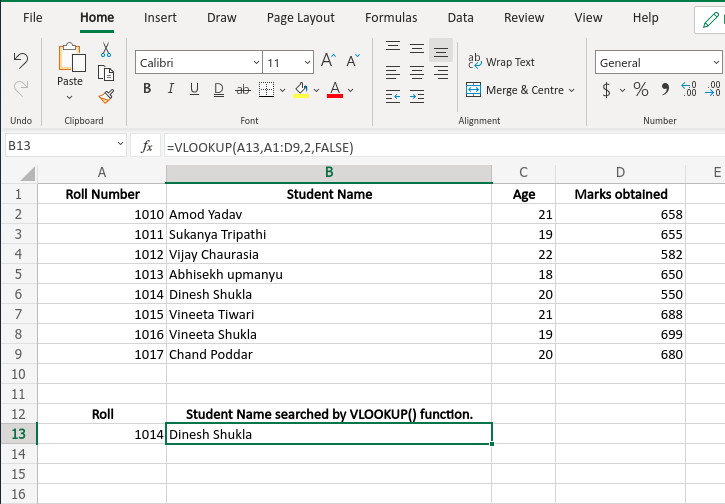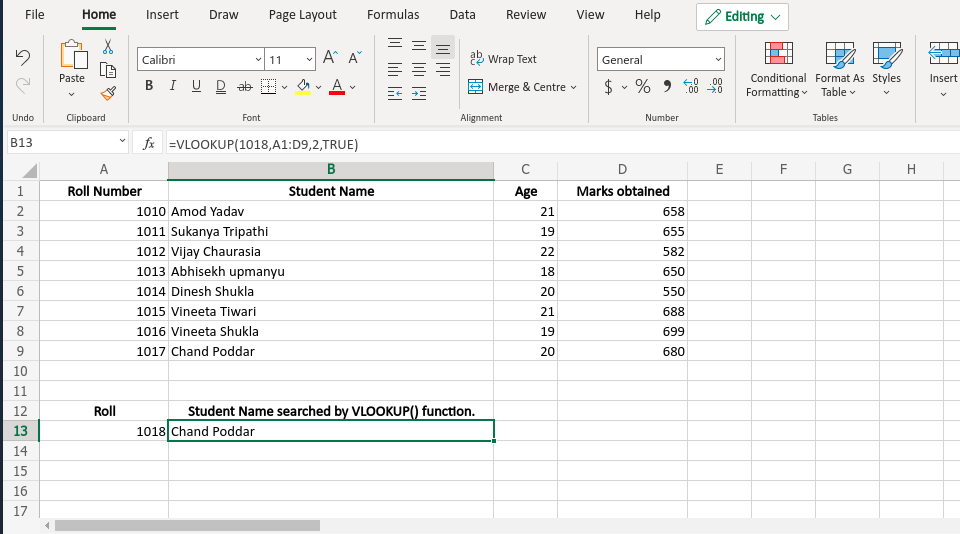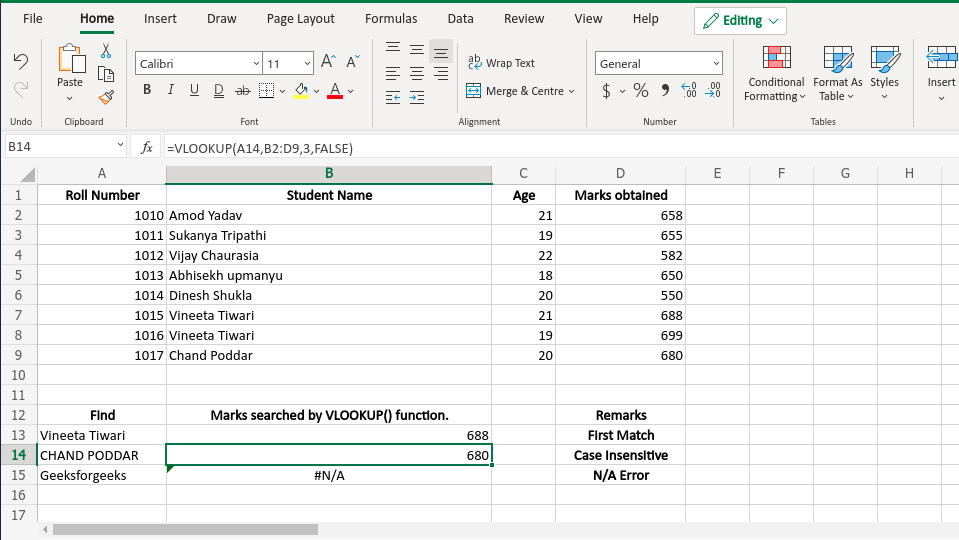Related Articles

# VLOOKUP Function in Excel With Examples

• Difficulty Level : Easy
• Last Updated : 15 May, 2021

Excel is widely used for data handling and almost everyone nowadays uses excel heavily. And at the same time, it is very user-friendly. Excel has several functions and formulas to make tedious jobs very easy. Like SUMIF() function VLOOKUP() is another very useful function widely used to ‘look up’ any data from a long table or a range by row. It supports approximate or exact matches based on one of its arguments being ‘True’ or ‘False’. This function also supports wildcards( ‘*’ and ‘?’) for partial matching.

Generally, VLOOKUP() function is used to find data from a table or a list or range. It has basically two features – Approximate match and Exact match.

Attention reader! Don’t stop learning now. If you are an Excel beginner (or an intermediate) and want to learn Excel, Geeksforgeeks brings the perfect course for you to start, Diving Into Excel

Note: User must set the LOOKUP -value in the first column of the selected table as VLOOKUP() always searches in the right of the value and returns the desired output.

```Syntax:
=VLOOKUP (lookup_value, table_array, col_index_num, [range_lookup])```

Arguments:

• lookup_value(Required): This is the lookup value that should be provided by the user and must be in the first column of the selected table.
• table_array(Required): This is the table where the lookup_value and our desired output both exist(Not necessarily the output exits all the time. In that case, it returns N/A error.). VLOOKUP() function uses this table_array argument to find the result.
• col_index_num(Required): This is the column number of the table_array where VLOOKUP() will search for its output. If it gets the result, it then returns the specific value.
• [range_lookup](optional): This argument is completely optional. The user may or may not provide this according to his need. This argument value may be True or False. If this is ‘True’, it means approximate match and if it’s ‘False’, it searches for an exact match. If nothing is specified by the user, by default this value is set to be ‘True’ and it searches for an approximate match.

Return Value:

This function searches the corresponding value of the given argument and returns the desired matched value from the table or range provided as an argument.

Examples:

• Exact Match:

Here, we will see an example of the exact match of the VLOOKUP() function.Example 1

• Approximate Match:

In this case, when an exact match is not found VLOOKUP() function will return the value of the next greater element. The following is the example.Example 2

Here, the 1018 roll number does not exist. So it returns the student name of roll number 1017(i.e the next greater element in the list).

• Example of First Match, Case Insensitive & N/A error:

This function always returns the first matched element. It is at the same time case-insensitive. And if we are looking for an element’s exact match while the element being absent in the table this function will show #N/A.

Note: This function always searches rightwards to the lookup_value.Example 3

My Personal Notes arrow_drop_up